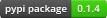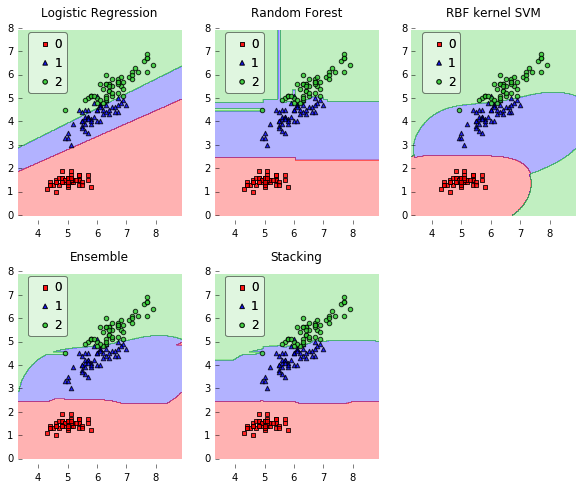# brew¶brew: A Multiple Classifier Systems API

This project was started in 2014 by Dayvid Victor and Thyago Porpino
for the Multiple Classifier Systems class at Federal University of Pernambuco.

The aim of this project is to provide an easy API for Ensembling, Stacking,
Blending, Ensemble Generation, Ensemble Pruning, Dynamic Classifier Selection,
and Dynamic Ensemble Selection.

## Example¶

```import numpy as np
import matplotlib.pyplot as plt
import matplotlib.gridspec as gridspec
import itertools

import sklearn

from sklearn.linear_model import LogisticRegression
from sklearn.svm import SVC
from sklearn.ensemble import RandomForestClassifier

from brew.base import Ensemble, EnsembleClassifier
from brew.stacking.stacker import EnsembleStack, EnsembleStackClassifier
from brew.combination.combiner import Combiner

from mlxtend.data import iris_data
from mlxtend.evaluate import plot_decision_regions

# Initializing Classifiers
clf1 = LogisticRegression(random_state=0)
clf2 = RandomForestClassifier(random_state=0)
clf3 = SVC(random_state=0, probability=True)

# Creating Ensemble
ensemble = Ensemble([clf1, clf2, clf3])
eclf = EnsembleClassifier(ensemble=ensemble, combiner=Combiner('mean'))

# Creating Stacking
layer_1 = Ensemble([clf1, clf2, clf3])
layer_2 = Ensemble([sklearn.clone(clf1)])

stack = EnsembleStack(cv=3)

stack.add_layer(layer_1)
stack.add_layer(layer_2)

sclf = EnsembleStackClassifier(stack)

clf_list = [clf1, clf2, clf3, eclf, sclf]
lbl_list = ['Logistic Regression', 'Random Forest', 'RBF kernel SVM', 'Ensemble', 'Stacking']

# Loading some example data
X, y = iris_data()
X = X[:,[0, 2]]

# Plotting Decision Regions
gs = gridspec.GridSpec(2, 3)
fig = plt.figure(figsize=(10, 8))

itt = itertools.product([0, 1, 2], repeat=2)

for clf, lab, grd in zip(clf_list, lbl_list, itt):
clf.fit(X, y)
ax = plt.subplot(gs[grd, grd])
fig = plot_decision_regions(X=X, y=y, clf=clf, legend=2)
plt.title(lab)
plt.show()
```### Features¶

• Ensembling, Blending and Stacking.
• Dynamic Classifier Selection: OLA, LCA, A Priori, A Posteriori.
• Dynamic Ensemble Selection: KNORA E and KNORA U.
• Ensemble Combination Rules: majority vote, min, max, mean and median.
• Ensemble Diversity Metrics: Entropy Measure E, Kohavi Wolpert Variance, | Q Statistics, Correlation Coefficient p, Disagreement Measure, Agreement Measure, Double Fault Measure.
• Ensemble Classifier Generators: Bagging, Random Subspace, SMOTEBagging, ICS-Bagging, SMOTE-ICS-Bagging.
• Ensemble Pruning: EPIC.
• Oversampling: SMOTE.

## Important References¶

• Kuncheva, Ludmila I. Combining pattern classifiers: methods and algorithms. John Wiley & Sons, 2014.
• Zhou, Zhi-Hua. Ensemble methods: foundations and algorithms. CRC Press, 2012.

## Dependencies¶

• Python 2.6+
• scikit-learn >= 0.14.1
• Numpy >= 1.3
• SciPy >= 0.7
• Matplotlib >= 0.99.1 (examples, only)
• mlxtend (examples, only)

## Feedback¶

If you have any suggestions or questions about brew feel free to email me at victor.dvro@gmail.com.

If you encounter any errors or problems with brew, please let me know! Open an Issue at the GitHub http://github.com/dvro/brew main repository.